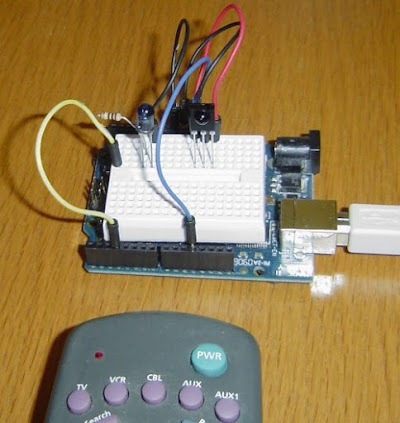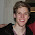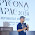Decoding an air conditioner control's checksum with differential cryptanalysis

Back in 2009 I wrote an Arduino library (IRRemote) to encode and decode infrared signals for remote controls. I got an email recently from someone wanting to control an air conditioner. It turns out that air conditioner remote controls are much complicated than TV remote controls: the codes are longer and include a moderately complex checksum. My reader had collected 35 signals from his air conditioner remote control, but couldn't figure out the checksum algorithm. I decided to use differential cryptanalysis to figure out the checksum, which was overkill but an interesting exercise. In case anyone else wants to decode a similar remote control, I've written up how I found the algorithm.My IR remote library can be used with the Arduino to send and receive signals. (This is not the air conditioner remote.)

The problem is to find a checksum algorithm that when given three bytes of input (left), computes the correct one byte checksum (right).

```10100001 10010011 01100011 => 01110111
10100001 10010011 01100100 => 01110001
10100001 10010011 01100101 => 01110000
10100001 10010011 01100110 => 01110010
10100001 10010011 01100111 => 01110011
10100001 10010011 01101000 => 01111001
10100001 10010011 01101001 => 01111000
10100001 10010011 01101010 => 01111010
10100001 10010011 01101011 => 01111011
10100001 10010011 01101100 => 01111110
10100001 10010011 01101101 => 01111111
10100001 10010011 01101110 => 01111100
10100001 10010011 01101111 => 01111101
10100001 10010011 01110001 => 01100100
10100001 10010011 01110010 => 01100110
10100001 10010011 01110011 => 01100111
10100001 10010011 01110100 => 01100001
10100001 10010011 01110101 => 01100000
10100001 10010011 01110111 => 01100011
10100001 10010011 01110111 => 01100011
10100001 10010011 01111000 => 01101001
10100001 10010011 01101000 => 01111001
10100001 00010011 01101000 => 11111001
10100001 00010011 01101100 => 11111110
10100001 10010011 01101100 => 01111110
10100001 10010100 01111110 => 01101011
10100001 10000010 01101100 => 01100000
10100001 10000001 01101100 => 01100011
10100001 10010011 01101100 => 01111110
10100001 10010000 01101100 => 01111100
10100001 10011000 01101100 => 01110100
10100001 10001000 01101100 => 01101100
10100001 10010000 01101100 => 01111100
10100001 10011000 01101100 => 01110100
10100010 00000010 11111111 => 01111110
```

The idea behind differential cryptanalysis is to look at the outputs resulting from inputs that have a small difference, to see what patterns emerge (details). Finding air conditioner checksums is kind of a trivial application of differential cryptanalysis, but using differential cryptanalysis provides a framework for approaching the problem. I wrote a simple program that found input pairs that differed in one bit and displayed the difference (i.e. xor) between the corresponding checksums. The table below shows the differences.

```000000000000000000000001 : 00000001
000000000000000000000010 : 00000010
000000000000000000000010 : 00000011
000000000000000000000100 : 00000100
000000000000000000000100 : 00000110
000000000000000000000100 : 00000111
000000000000000000001000 : 00001100
000000000000000000001000 : 00001110
000000000000000000001000 : 00001111
000000000000000000010000 : 00010000
000000000000100000000000 : 00001000
000000000001000000000000 : 00011000
000000001000000000000000 : 10000000
```

The first thing to notice is that changing one bit in the input causes a relatively small change in the output. If the checksum were something cryptographic, a single bit change would entirely change the output (so you'd see half the bits flipped on average). Thus, we know we're dealing with a simple algorithm.

The second thing to notice is the upper four bits of the checksum change simply: changing a bit in the upper four bits of an input byte changes the corresponding bit in the upper four bits of the output. This suggests that the the three bytes are simply xor'd to generate the upper four bits. In fact, my reader had already determined that the xor of the input bytes (along with 0x20) yielded the upper four bits of the checksum.

The final thing to notice is there's an unusual avalanche pattern in the lower four bits. Changing the lowest input bit changes the lowest checksum bit. Changing the second-lowest input bit changes the second-lowest checksum bit and potentially the last checksum bit. Likewise changing the fourth-lowest input bit changes the fourth-lowest checksum bit and potentially the bits to the right. And the change pattern always has 1's potentially followed by 0's, not a mixture. (1100, 1110, 1111)

What simple operation has this sort of avalanche effect? Consider adding two binary numbers. If you change a high-order bit of an input, only that bit will change in the output. If you change a low-order input bit, the low-order bit of the output will change. But maybe there will be a carry, and the next bit will change. And if there's a carry from that position, the third bit will change. Likewise, changing a bit in the middle will change that bit and potentially some of the bits to the left (due to carries). So if you change the low-order bit, the change in the output could be 0001 (no carry), or 0011, or 0111, or 1111 (all carries). This is the same pattern seen in the air conditioner checksums but backwards. This raises the possibility that the checksum is using a binary sum, but we're looking at the bits backwards.

So I made a program that reversed the bits in the input and output, and took the sum of the four bits from each byte. The output below shows the reversed input, the sum, and the 4-bit value from the correct checksum. Note that the sum and the correct value usually add up to 46 or 30 (two short of a multiple of 16). This suggested that the checksum is `(-sum-2) & 0xf`.

```110001101100100110000101 32 14
001001101100100110000101 22 8
101001101100100110000101 30 0
011001101100100110000101 26 4
111001101100100110000101 34 12
000101101100100110000101 21 9
100101101100100110000101 29 1
010101101100100110000101 25 5
110101101100100110000101 33 13
001101101100100110000101 23 7
101101101100100110000101 31 15
011101101100100110000101 27 3
111101101100100110000101 35 11
100011101100100110000101 28 2
010011101100100110000101 24 6
110011101100100110000101 32 14
001011101100100110000101 22 8
101011101100100110000101 30 0
111011101100100110000101 34 12
111011101100100110000101 34 12
000111101100100110000101 21 9
000101101100100110000101 21 9
000101101100100010000101 21 9
001101101100100010000101 23 7
001101101100100110000101 23 7
011111100010100110000101 17 13
001101100100000110000101 15 0 *
001101101000000110000101 19 12 *
001101101100100110000101 23 7
001101100000100110000101 11 3
001101100001100110000101 12 2
001101100001000110000101 12 3 *
001101100000100110000101 11 3
001101100001100110000101 12 2
111111110100000001000101 23 7
```

That formula worked with three exceptions (marked with asterisks). Studying the exceptions showed that adding in byte 1 bit 3 and byte 2 bit 7 yielded the correct answer in all cases.

Conclusion

Putting this together yields the following algorithm (full code here):

```inbytes = map(bitreverse, inbytes)
xorpart = (inbytes ^ inbytes ^ inbytes ^ 0x4) & 0xf
sumpart = (inbytes >> 4) + (inbytes >> 4) + (inbytes >> 4) +
(inbytes & 1) + ((inbytes >> 3) & 1) + 1
sumpart = (-sumpart) & 0xf
result = bitreverse((sumpart << 4) | xorpart)
```

Is this the right formula? It gives the right checksum for all the given inputs. However, some bits never change in the input data; in particular all inputs start with "101000", so there's no way of knowing how they affect the algorithm. They could be added to the sum, for instance, replacing the constant `+1`. The constant 0x4 in the xor also makes me a bit suspicious. It's quite possible that the checksum formula would need to be tweaked if additional input data becomes available.

I should point out that determining the checksum formula is unnecessary for most air conditioning applications. Most users could just hard-code the checksums for the handful of commands they want to send, rather than working out the general algorithm.

Thanks to Antonio Martinez Lavin, maker of the Cuby air conditioner controller for posing this problem. If you want to use my IR library, it is on GitHub. I'm no longer actively involved with the library, so please post issues on the GitHub repository rather than on this blog post. Thanks to Rafi Kahn, who has taken over library maintenance and improvement.

1.Thanks a lot, that will come in handy in future!

Could you make the height of the <pre> blocks larger, and/or resizable? Right now, viewing the article on Safari at least, the blocks with the binary patterns only show 4 lines at once, which is a bit cumbersome to read.

2.Wow, great write up. I went through a similar process reverse engineering 433 MHz RF codes for some motorized blinds! https://nickwhyte.com/post/2017/reversing-433mhz-raex-motorised-rf-blinds/

3.This comment has been removed by the author.

4.Really interesting work. I wonder if it can be implemented in other things. I don't know the standard used for package delivery code in my country. Can I use this to decode the pattern too?

5.When you compute sumpart, you basically compute some 'x' from input bits, then sumpart = (-x -1) & 0xf. -x - 1 is equivalent to ~x. I guess that is how it was implemented

6.That's what we engineers do, Instead of getting up and changing the temperature, we reverse engineer the protocol for said air conditioner. Then we use a microcontroller with a custom interface and a IR light to substitute for a real remote. A lot easier than getting up and doing it manually!

7.Great information and I am very much thankful to you for this blog. Kindly visit my page too: kenstar split ac review